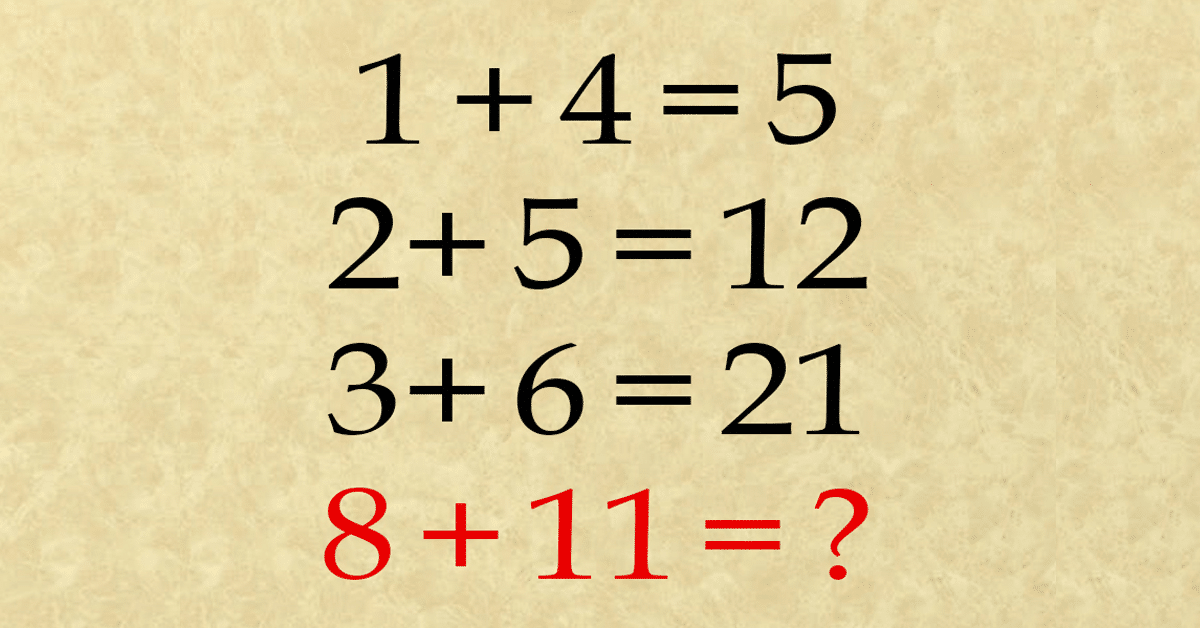# Can you solve this tough number puzzle? It’s leaving so many people stumpedThis number puzzle isn’t the norm for everyone but having a go at it will certainly give your gray matter the work out it needs.

Can you work it out?The first number sentence is obvious: 1 + 4 is definitely 5 but then the next two lines don’t follow suit.

In working out the next two number sentences and why the answer is what it is you will certainly ace the last sentence.

The number pattern

Take the answer to the previous number equation and add it to the number of the next equation.

In the case of the first equation, there is no previous equation, and so you would add zero to the first equation (0 + 1 + 4), which gives you 5.

The same pattern works for the second and third equations. Apply this rule to the last equation, and you will get the solution.The answer to the puzzle is 40! Did you get it right? It’s easy when you know how.